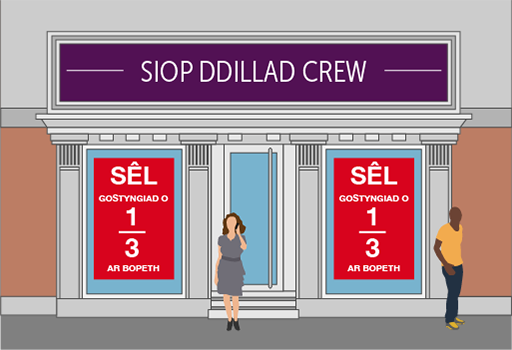Everyday maths 1 (Wales)

Start this free course now. Just create an account and sign in. Enrol and complete the course for a free statement of participation or digital badge if available.

Free course

# 3.4 Fractions of amounts

Have a look at the following examples, which demonstrate how you would find the fraction of an amount.

## Example: Finding fractions

### SaleFigure 18 Fractions in a sale

Say you go into a shop to buy a dress. Usually it would cost £90, but today it’s in the ‘ off’ sale. How much would you get off?

### Method

The basic rule for finding a unit fraction of an amount is to divide by how many parts there are (the number on the bottom of the fraction) and multiply the result by the number at the top of the fraction. To work out off £90 is the same as:

£90 ÷ 3 = £30

The sum £30 × 1 = £30, so you would get £30 off.

### Survey

In a survey, of respondents said that they would like to keep the pound as the currency of the UK. If 800 people were surveyed, how many people wanted to keep the pound?

### Method

Again, to find a fraction of an amount you need to divide by the number at the bottom of the fraction and then multiply that result by the number at the top of the fraction:

To answer this you need to first work out what of 800 people is.

of 800 = 800 ÷ 4 = 200

Then use the numerator (the top of the fraction) to work out how many of those unit fractions are needed:

of 800 = 3 × 200 = 600

So 600 people wanted to keep the pound.

## Activity 22: Paying in instalmentsFigure 19 How much would an extension cost?

A family plans to have its kitchen extended.

The cost of this project is £12 000.

The builder they have chosen to carry out this job has asked for the money to be paid in stages:

1. of the money to be paid before starting the project.
2. of the money to be paid a month later.
3. The remainder to be paid when the extension has been built.

How much is the builder asking for during Stage 1 and Stage 2?

To work out of £12 000 you need to divide £12 000 by 5.

• 12 000 ÷ 5 = 2 400

Now multiply by the number on the top of the fraction:

• 2 400 × 1 = £2 400

So at Stage 1 the builder will need £2 400.

To work out of £12 000 you need to first work out of £12 000. To do this you need to divide £12 000 by 3.

• 12 000 ÷ 3 = 4 000

You now need to work out of £12 000 so you multiply by the number on the top of the fraction:

• 4 000 × 2 = 8 000

So at Stage 2 the builder will need £8 000.

## Summary

In this section you have learned how to:

• find equivalencies in fractions
• order and compare fractions
• find the fraction of an amount.

The skills listed above can be used when you are shopping and trying to get the best deal, or when you are splitting a cake or a pizza, say, into equal parts.

It’s important to be able to compare fractions, decimals and percentages in real-life situations. You’ll be looking at percentages later, but first you can look at decimals.

FSM_1_CYMRU